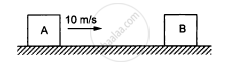Advertisement Remove all ads

# The Blocks Shown in Figure Have Equal Masses. the Surface of a is Smooth but that of B Has a Friction Coefficient of 0.10 with the Floor. Block a is Moving at a Speed of 10 M/S Towards - Physics

Numerical

The blocks shown in figure have equal masses. The surface of A is smooth but that of Bhas a friction coefficient of 0.10 with the floor. Block A is moving at a speed of 10 m/s towards B which is kept at rest. Find the distance travelled by B if (a) the collision is perfectly elastic and (b) the collision is perfectly inelastic.Advertisement Remove all ads

#### Solution

Given,
Speed of the block A = 10 m/s
The block B is kept at rest.
Coefficient of friction between floor and block B, μ = 0.10

Lets v1 and v2 be the velocities of A and B after collision respectively.

(a) If the collision is perfectly elastic, linear momentum is conserved.

Using the law of conservation of linear momentum, we can write:

$m u_1 + m u_2 = m v_1 + m v_2$

$\Rightarrow 10 + 0 = v_1 + v_2$

$v_1 + v_2 = 10 . . . \left( 1 \right)$

$\text{ We know,}$

$\text{ Velocity of separation } \left( \text{ after collision }\right) = \text{ Velocity of approach } \left( \text{ before collision } \right )$

$v_1 - v_2 = - ( u_1 - v_2 )$

$\Rightarrow v_1 - v_2 = - 10 . . . \left( 2 \right)$

$\text{ Substracting equation (2) from (1), we get: }$

$2 v_2 = 20$

$\Rightarrow v_2 = 10 \text{ m/s }$
The deceleration of block B is calculated as follows:

Applying the work energy principle, we get:

$\left( \frac{1}{2} \right) \times m \times (0 )^2 - \left( \frac{1}{2} \right) \times m \times v^2 = - m \times a \times s_1$

$\Rightarrow - \left( \frac{1}{2} \right) \times (10 )^2 = - \mu g \times s_1$

$\Rightarrow s_1 = \frac{100}{2 \times 1 \times 10} = 50 \text{ m }$

(b) If the collision is perfectly inelastic, we can write:

$m \times u_1 + m \times u_2 = (m + m) \times v$

$\Rightarrow m \times 10 + m \times 0 = 2m \times v$

$\Rightarrow v = \left( \frac{10}{2} \right) = 5 \text{ m/s }$
The two blocks move together, sticking to each other.
∴ Applying the work-energy principle again, we get:

$\left( \frac{1}{2} \right) \times 2m \times (0 )^2 - \left( \frac{1}{2} \right) \times 2m \times (v )^2 = 2m \times \mu g \times s_2$

$\Rightarrow \frac{(5 )^2}{0 . 1 \times 10 \times 2} = s_2$

$\Rightarrow s_2 = 12 . 5$  m

Concept: Momentum Conservation and Centre of Mass Motion
Is there an error in this question or solution?
Advertisement Remove all ads

#### APPEARS IN

HC Verma Class 11, 12 Concepts of Physics 1
Chapter 9 Centre of Mass, Linear Momentum, Collision
Q 58 | Page 163
Advertisement Remove all ads
Advertisement Remove all ads
Share
Notifications

View all notifications

Forgot password?